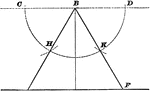### Construction Of Equilateral Triangle

Illustration used to show how to draw an equilateral triangle when given the altitude.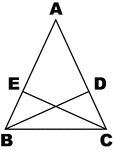### Altitudes Drawn from Base Angles of Isosceles Triangles

Illustration to show altitudes drawn from base angles of an isosceles triangle.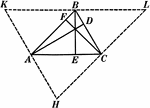### Altitudes In A Triangle

Illustration used to show "The altitudes of a triangle are concurrent."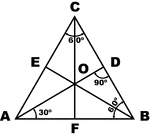### Equilateral Triangle

Sketch of equilateral triangle with bisectors, medians, and altitudes.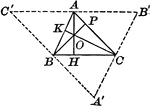### Orthocenter of Triangle

Illustration to show altitudes in a triangle. It is known as the orthocenter.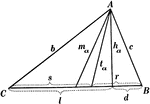### Parts Of A Triangle

Illustration used to show the various parts of a triangle: sides, angles, medians, altitudes, bisectors,…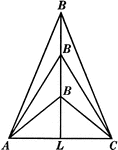### 3 Isosceles Triangles Wit Equal Bases

Illustration of three isosceles triangles with the same base AC but varying heights (as B gets farther…### Similar Triangles With Altitudes Drawn

Illustration that shows two similar triangles with altitudes drawn.